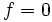# Second cohomology group for nontrivial group action of Z4 on Z4

This article gives information about the second cohomology group for a specified nontrivial action of the group cyclic group:Z4 on cyclic group:Z4. The elements of this classify the group extensions with cyclic group:Z4 as an abelian normal subgroup and cyclic group:Z4 the corresponding quotient group.
The value of this cohomology group is cyclic group:Z2.
Get more specific information about cyclic group:Z4 |Get more specific information about cyclic group:Z4|View other constructions whose value is cyclic group:Z2

## Description of the group

Let$G$ be cyclic group:Z4 with generator$g$ and$A$ be cyclic group:Z4 with generator$a$. Note that the automorphism group$\operatorname{Aut}(A)$ is isomorphic to cyclic group:Z2, with its non-identity element being the inverse map.

Consider the homomorphism$\varphi:G \to \operatorname{Aut}(A)$ that sends$g$ to the inverse map of$A$. Accordingly,$\varphi(g^2)$ is the identity map and$\varphi(g^3)$ is also the inverse map of$A$.

We are interested in the second cohomology group for the action of$G$ on$A$, i.e., the group:$\! H^2_\varphi(G,A)$

The cohomology group is isomorphic to cyclic group:Z2.

## Elements

We consider here the two cohomology classes, with representative cocycles for each. For simplicity, we choose a representative cocycle that is a normalized 2-cocycle, i.e., if either of the inputs is the identity element of$G$, the output is the identity element of$A$.

Cohomology class type Number of cohomology classes Representative cocycle Corresponding group extension GAP ID second part (order is 16) Base characteristic in whole group?
trivial 1$f = 0$ everywhere nontrivial semidirect product of Z4 and Z4 3 No
nontrivial 1$f(g^i,g^j) = a^2$ if$i + j \ge 4$, where we choose$0 \le i,j \le 3$ M16 6 Yes